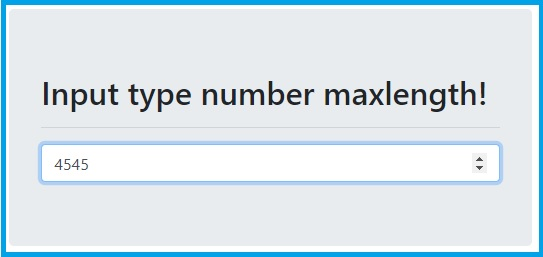# Input type number maxlength not working

Last updated 3 years, 7 months ago | 14307 views# HTML | Input type number maxlength not working

The "maxlength" attribute of an input field is used to limit the length entered in the input field. but it works only if the type of input field is text, password, search, tel, email, and URL.

check out the link for more details: MDN's documentation

So maxlength attribute does not work for the input type number, it is ignored. but using jquery it can work fine.

The jquery code for make maxlength attributes workable for input type number.

```\$(".num").keypress(function() {
if (\$(this).val().length == \$(this).attr("maxlength")) {
return false;
}
});```

In the above code "keypress()" function is used so that it can track whenever the user presses any key. then there is a "if()" condition in which "\$(this).val().length" keep the current value in the input field and "\$(this).attr("maxlength")" take the value of maxlength attribute defined in input field. so once the condition satisfy it return false. hence no more value can be accepted.

## Here seen an example with complete code

In this example, there is an input field with a type number and maxlength attribute value is 4. The input field can accept only four-digit. user can change the number but can not add more than 4 digits.

``````<!DOCTYPE html>
<html lang="en">

<title>Input type number maxlength not working</title>

<!-- Latest compiled and minified CSS -->

<!-- jQuery library -->

<!-- Script to limit max length in input type number -->
<script>
\$(".num").keypress(function() {
if (\$(this).val().length == \$(this).attr("maxlength")) {
return false;
}
});
});
</script>

<body>
<div class="row">
<div class="col-md-6 offset-md-3">
<div class="jumbotron mt-5">
<h2 class="display-6">Input type number maxlength!</h2>
<hr>
<input type="number" class="form-control num" maxlength="4" />
</div>
</div>
</div>
</body>

</html>
``````